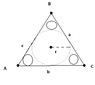# I am a new member and i need a little help,if possible

• sutupidmath

#### sutupidmath

It is geometry.

In a isosceles triangle is i nscribed a circle. Three more circles are inscribed in that triangle so that every circle touches the big circle exactly at one point and the two sides of the triangle exactly at one point. So the problem is how to set the ration between the surface of the big circle and the sum of surface of the three smaller circles inscribed in that triangle.

#### Attachments

•inscribed_1.GIF
1.4 KB · Views: 481

One important thing here is that the center of the incribed circle is at the intersection of the bisectors. You can construct the smaller circles by treating them as being inscribed into smaller triangles. Work around that.

One last tip: one of the side of the "smaller triangles" will be tangent to the bigger circle.

Last edited:
Thankyou for the information, however i have tried that, and many other approches to it, however the problem is that if you could be more specific about how to express the radius of the larger circle as a function of the smaller circles' radiis? What should i use?
I have tried to use the pythagorian theorem and also the similarities abd ratios between the smaller circles that we can construct around smaller circles and the big ones, however i got nowere. If you could give me a more specific hint, so i could know exactly how to approach it i would appreciate it. I have given quite an effort to this problem.

thankyou guys

Hummm okay, but my approach requires a bit of analysis. I'm not even sure there is a constant ratio.

1. Start by placing your triangle on a XY plane, with the lower left vertex at the origin. Let the two lower angles be q and the top angle p. Now the coordinates of each vertex can be found with trigonometry. We have A = (0,0), B = a (cos q, sin q) and C = (b, 0).

2. Find the inscribed circle by finding the functions of two bisectors. At B, the bisector is x = a*cos q, and at A, y = tan (q/2). Now obviously, the intersection would be at [ a*cos q , tan (1/2 a*cos q) ].

3. Since we are dealing with an isosceles triangle, the circle touches side b at x = b/2 = a*cos q. This also means that the radius of the circle is tan (1/2 a*cos q).

4. Now, that you have the radius of the circle, you want to find the radius of the smaller circles. Say we take the circle that is close to A. We want it to be tangent to the bigger circle. To do so, we have to construct a smaller triangle such that it has one side tangent to the circle AND that this side is perpendicular to the bisector of A. This requires some light calculus.

... Now can you finish the job?

what did you actually mean by * ?

Multiplies...